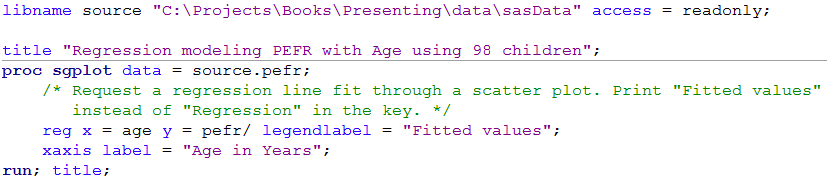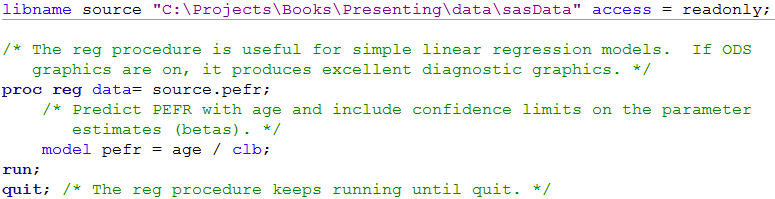﻿ Chapter 9: Analysing relationships between variables using SAS

# Chapter 9: Analysing relationships between variables using SAS

### Box 9.3 Presenting the results for Pearson’s correlation### Figure 9.1, Figure 9.2 and Box 9.3 Code### Figure 9.3 Scatterplots for several variables### Code Figure 9.3### Box 9.5 Presenting correlations between several variables### Code Figure 9.4 and Box 9.5### Figure 9.5 Presenting scatterplots (a) with skewed data and (b) where data are transformed### Figure 9.5 Code### Figure 9.6 Output for a rank test### Figure 9.6 Code### Figure 9.7 Scatterplot of two variables with linear regression line### Figure 9.7 Code### Figure 9.8 Output for simple regression### Figure 9.8 Code### Box 9.11 Presenting the results for several predictor variables### Box 9.11 Code### Box 9.13 Presenting the results of a regression model that uses a log transformation of the outcome variable### Box 9.13 CodeSAS can not easily produce this output

### Figure 9.9 Scatterplot of two variables with linear regression line and 95% confidence intervals### Box 9.14 and Figure 9.9 Code